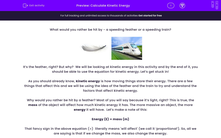# Calculate Kinetic Energy

In this worksheet, students will learn how to use the kinetic energy equation, as well as deepen their understanding of kinetic energy.Key stage:  KS 4

Year:  GCSE

GCSE Boards:   AQA, AQA Trilogy, AQA Synergy, OCR 21st Century, OCR Gateway, Pearson Edexcel, Eduqas,

Curriculum topic:   Energy, Movement and Interactions, Explaining Motion, Conservation of Energy

Difficulty level:#### Worksheet Overview

What would you rather be hit by - a speeding feather or a speeding train?It’s the feather, right? But why?  We will be looking at kinetic energy in this activity and by the end of it, you should be able to use the equation for kinetic energy. Let’s get stuck in!

As you should already know, kinetic energy is how moving things store their energy. There are a few things that affect this and we will be using the idea of the feather and the train to try and understand the factors that affect kinetic energy.

Why would you rather be hit by a feather? Most of you will say because it’s light, right? This is true, the mass of the object will affect how much kinetic energy it has. The more massive an object, the more energy it will have.  Let’s make a note of this:

Energy (E) ∝ mass (m)

That fancy sign in the above equation (∝)  literally means 'will affect' (we call it ‘proportional’). So, all we are saying is that if we change the mass, we also change the energy.

Let’s change the situation a little bit now. The train is moving at 0.01 m/s and the feather is moving at 1,000 m/s. Which one would you rather be hit by now? Did you say the train? That’s because speed (or velocity) also has an effect on the kinetic energy. Let’s add this to our proportionality equation:

Energy (E) ∝ mass (m) x velocity (v)

However, it isn’t as simple that…scientists found that the mass doesn’t make as much difference as the velocity. In fact, the mass needs to be halved. They also found that the velocity makes a big difference to the amount of energy. They found that the velocity squared gives us energy.So how do you use this equation? Simple! Let’s go through an example together.

Jim runs at 3 m/s and has a mass of 60 kg. What is Jim’s kinetic energy?

Step 1  Find the values in the question and highlight them:

Jim runs at 3 m/s and has a mass of 60 kg. What is Jim’s kinetic energy?

Step 2  Write out the values next to their symbols:

mass = 60 kg

velocity = 3 m/s

Step 3  Halve the mass and square the speed:

m = 30 kg

v = 9 m/s

Step 4  Put these into the equation:

E = 30 x 9

Step 5  Put these into your calculator and press =

E = 270 J

Don’t forget the unit of energy is the joule (J).

Now it's time to have a go at some questions.

### What is EdPlace?

We're your National Curriculum aligned online education content provider helping each child succeed in English, maths and science from year 1 to GCSE. With an EdPlace account you’ll be able to track and measure progress, helping each child achieve their best. We build confidence and attainment by personalising each child’s learning at a level that suits them.

Get started